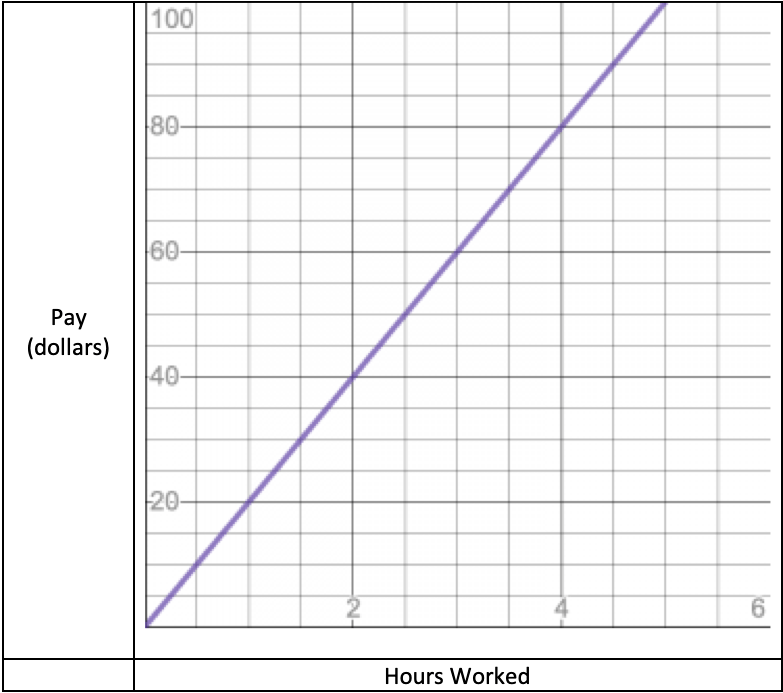# Comparing Data in Different Formats

This post about comparing data in different formats is part of a series of posts to help you prepare for the Advanced Algebra and Functions part of the Accuplacer test.

## QuestionLiam’s Pay

This graph shows how much Liam gets paid as a function of the number of hours he works. Sarah, on the other hand, is paid according to the formula S=(75/4)h, where S represents Sarah’s pay and h represents the number of hours she has worked.

Is Liam’s rate of pay more or less than Sarah’s, and by how much?

## Solution

You’re being asked to compare data that measure similar things but are given to you in different formats.

The trick is to convert one of those expressions into the same format as the other. Or, if you can’t do that, then convert both to some third format.

P=(75/4)h looks . . . almost straightforward. That fraction may be inconvenient. 75/4 = 18.75, so let’s say S = 18.75h represents Sarah’s rate of pay.

Now look at the graph. Can you represent Liam’s pay as something like L=kh, where k is Liam’s rate of pay? Since the graph goes through the origin, its equation will be of the form L=something*and “something” is the slope. To find it from the graph, pick two points on the line that are easy to read, and calculate. The origin — with coordinates (0, 0) — looks easy. And the point (2, 40) doesn’t look bad. The slope is the change in y divided by the change in for those two points:

So Liam’s pay is L=20h.

You’re asked for the difference between Liam’s rate of pay and Sarah’s. That is

Answer: Liam gets paid \$1.25 per hour more than Sarah.

For more about relating a line’s equation to its graph, see “Finding a Line’s Equation from the Graph of Its Perpendicular.

This question is similar to question number 5 in the sample questions for the Accuplacer Advanced Algebra and Functions test.

0 replies